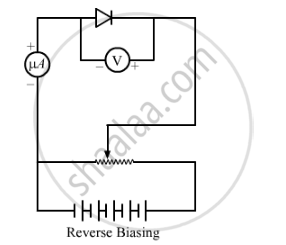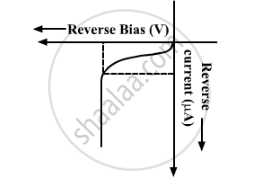# Using the Necessary Circuit Diagrams, Show How the V-I Characteristics of a p-n Junction Are Obtained in Reverse Biasing - Physics

Using the necessary circuit diagrams, show how the V-I characteristics of a p-n junction are obtained in

Reverse biasing

How are these characteristics made use of in rectification?

#### Solution

p-n junction diode under reverse biasPositive terminal of battery is connected to n-side and negative terminal to p-side.

Reverse bias supports the potential barrier. Therefore, the barrier height increases and the width of depletion region also increases.

Due to the majority carriers, there is no conduction across the junction. A few minority carriers cross the junction after being accelerated by high reverse bias voltage.

This constitutes a current that flows in opposite direction, which is called reverse current.

The V-I characteristics of p-n junction diode in reverse bias is shown below:p-n junction diode is used as a half-wave rectifier. Its working is based on the fact that the resistance of p-n junction becomes low when forward biased and becomes high when reverse biased. These characteristics of diode is used in rectification.

Concept: Special Purpose P-n Junction Diodes
Is there an error in this question or solution?